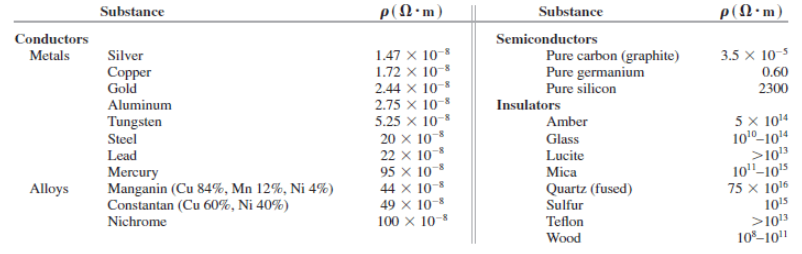# Problem: Pure silicon contains approximately 1.0 x 1016 free electrons per cubic meter.Resistivities at Room Temperature (20 °C)(a) Referring to the table above, calculate the mean free time for silicon at room temperature.(b) Your answer in part (a) is much greater than the mean free time for copper. Why, then, does pure silicon have such a high resistivity compared to copper?

###### FREE Expert Solution

(a)

Mean free time, Tλ

$\overline{){{\mathbf{T}}}_{{\mathbf{\lambda }}}{\mathbf{=}}\frac{\mathbf{m}}{\mathbf{n}{\mathbf{e}}^{\mathbf{2}}\mathbf{\rho }}}$

93% (32 ratings)###### Problem Details

Pure silicon contains approximately 1.0 x 1016 free electrons per cubic meter.

Resistivities at Room Temperature (20 °C)(a) Referring to the table above, calculate the mean free time for silicon at room temperature.
(b) Your answer in part (a) is much greater than the mean free time for copper. Why, then, does pure silicon have such a high resistivity compared to copper?